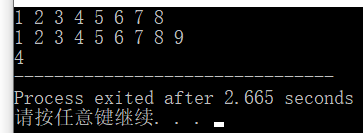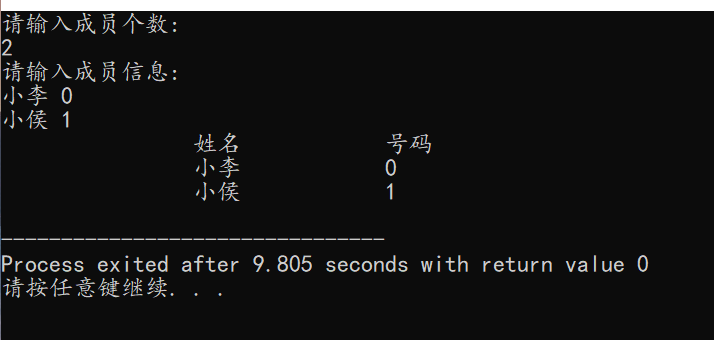• C++定义链表类模板
2021-11-08 17:14:19
声明一个 Student 结构体或者类，数据成员是学号(int)，姓名(string)和成绩
(float)，然后定义一个 list 链表类对象，追加 10 个 Student 对象到 list 对象
里面，然后将自己的数据（学号姓名和成绩）插入到其中的第 6 个位置。用
迭代器遍历整个 list 对象，输出全部数据项的值。
#include<iostream>
#include<list>
using namespace std;
struct Student
{
int number;
string name;
float score;
};
int main()
{
int i;
list<Student> List;
Student stu={{0,"aa",98.1},{1,"bb",96.3},{2,"cc",95.2},{3,"dd",97.3},{4,"ee",94.2},{5,"ff",95.9},{6,"gg",91.9},{7,"hh",96.5},{8,"ii",91.9},{9,"jj",91.5}};
for(i=0;i<10;i++)
List.push_back(stu[i]);
list<Student>::iterator iter=List.begin();
for(i=0;i<5;i++)
iter ++;
List.insert(iter,{11,"jz",99.9});
for(iter=List.begin();iter!=List.end();iter++)
cout<<(*iter).number<<","<<(*iter).name<<","<<(*iter).score<<endl;
return 0;
}list 数据结构
更多相关内容
• C++链表类 模板类 #include #include #include "LinkedList.h" using namespace std; template Node<T> *LinkedList<T>::GetNode(const T& item, Node* ptrNext) //生成新结点 { Node<T> *p; p = new Node...
• ## C++链表类模板

千次阅读 2020-01-28 12:12:41
C++链表类模板 链表（list），即双向链表容器，它不支持随机访问，访问链表元素要指针从链表的某个断点开始，插入和删除操作所花费的时间是固定的，和该元素在链表中的位置无关。list在任何位置插入和删除动作都很快...

# C++链表类模板

链表（list），即双向链表容器，它不支持随机访问，访问链表元素要指针从链表的某个断点开始，插入和删除操作所花费的时间是固定的，和该元素在链表中的位置无关。list在任何位置插入和删除动作都很快，不像vector只能在末尾操作。

#### 创建list链表类模板的方法：

#include<list>		//调用list头文件
...
...
list<type>l;			//定义一个list
//list表示声明一个链表，<type>表示链表的类型，l表示链表名。


#### list对象主要成员函数说明：

只是一些常用的，其它还有很多list函数。

函数说明
begin返回指向链表第一个元素的迭代器
end返回指向链表最后一个元素的迭代器
size返回链表的大小
clear删除链表中所有元素
erase(start,end)删除迭代器从start到end范围内的向量
erase(i)删除迭代器第i个元素
insert(i,x)把值x插入到由迭代器指明的第i个位置
insert(i,start,end)把迭代器从start到end范围的元素插入到指明的第i个位置
insert(i,n,x)把x的n个副本插入到由迭代器指明的第i个位置
push_back(x)将值x放到链表末尾
push_front(x)将值x放到链表首部
pop_back删除链表最后一个元素
pop_front删除链表第一个元素
resize(n,x)设置链表大小为n，初始值为x
reverse颠倒元素的顺序
swap(list)交换两个链表的内容

可以发现，list链表所支持的操作与vector向量很相近，但这些操作的实现原理不尽相同，执行效率也不一样。list双向链表的优点是插入元素的效率很高，缺点是不支持随机访问，也就是说，链表无法像数组一样通过索引来访问，形如：

list<int>l;
l=4;			//错误
cout<<l;		//错误


链表无法通过索引进行赋值和输入输出。

关于vector向量的学习————》》C++向量类模板

#### 迭代器

对list双向链表各个元素的访问，通常使用迭代器。
迭代器定义方法：

list<int>::iterator it=l.begin();


定义了一个迭代器it指向双向链表 l 的开始指针。

#### list双向链表与迭代器的入门应用

源代码：

#include<iostream>
#include<list>		//list头文件
using namespace std;
int main(){

list<int>l;					//定义链表l
int a={1,3,8,6,7,2,5,4};	//定义数组a
l=list<int>(a,a+8);			//将数组a赋值给l
l.sort();					//排序

list<int>::iterator it1=l.begin();	//定义迭代器it1指向双向链表 l 的开始指针
for(;it1!=l.end();it1++)
cout<<*it1<<" ";				//通过变更it1的指向遍历输出l中的所有元素
cout<<endl;

l.insert(l.end(),9); 			//将9插入到l的末尾
list<int>::iterator it2=l.begin();
for(;it2!=l.end();it2++)
cout<<*it2<<" ";
cout<<endl;

list<int>::iterator it3=l.begin();
cout<<*it3+3;		//通过迭代器加减的方式取代索引输出

return 0;
}


值得一提的是，循环终止的条件是it!=l.end()而非人们惯用的it<l.end()

结果图：展开全文指针 数据结构 c语言
• C++链表类模版 //构造函数 //创建n个元素的列表 //拷贝构造 //释放链表 //析构函数（虚） //输出链表 //用t替换掉当前结点内容 //重载赋值运算符= //获取当前链表的长度或结点数 // 当前结点位置：第 n （从 0 起）...
• 1. 请创建一个数据类型为T的链表类模板List，实现以下成员函数： 1） 默认构造函数List()，将该链表初始化为一个空链表（10分） 2） 拷贝构造函数List(const List<T>& list)，根据一个给定的链表构造当前链表（10...
• c++链表类模板的应用

类模板都是以template开头写起，模式如下：

template<class type>  //其中class 是固定格式  type 可以指向任何一个数据的类型 如double等。
//函数模板可如下写出

type 函数名称（参数1，参数2）

//类模板如下

class 类名
{
数据成员

成员函数
}；

<>内为模板参数，模板参数主要分为两种：

一种是模板类型参数，另一种是模板非类型参数.

当模板被调用时，模板中的代码才会被执行。也就相当于一个实例，称为模板类或模板函数.

创建一个链表类模板步骤如下

1.先创造一个类模板

template<class type>
class clist  //类模板
{
private:                      //类中的私有成员

int m_nodesum;            //节点数量
public：
clist（）                 //构造函数
{
m_nodesum = 0;
//给每个数据初始化
}

type *movetrial()         //获取尾结点的函数
{
for(int i = 1; i < m_nodesum; i++)
{
ptemp->ptemp->next;      //next指向下一个节点的指针，在后续的类中出现。
}
return ptemp;

}

{
if(m_nodesum == 0)    //判断链表是否为空
{
}
else
{
type *ptrail = movetrial();    //获取尾结点的位置
ptrail->next = pnode;
}
m_nodesum++;    //每次多一个数据存储时节点数加一
}

void passlist()
{
if(m_nodesum > 0)
{
printf("\t\t姓名\t\t号码\n");
printf("\t\t%s\t\t%d\n",ptmp->name,ptmp->num);
for(int i = 1; i < m_nodesum; i++)
{
ptmp=ptmp->next;    //获取下一个节点
printf("\t\t%s\t\t%d\n",ptmp->name,ptmp->num);
}
}
}

~clist()    //类中的析构函数，将所有的数据都进行删除处理
{
if(m_nodesum > 0)
{
type *t=NULL;
for(int i = 0; i < m_nodesum; i++)
{
t = pdelete->next;    // 保存链表后一个未被删除的数据
delete pdelete;    //释放当前节点
pdelete = t;
}
m_nodesum = 0;
pdelete = NULL;
t = NULL;
}
}
};

链表的类模板完成后，要出现一个参数类来代替其中的type。并起到可以保存数据的作用，可以使用类或者结构体都可以完成本操作。（其中结构体和类的一个区别是：结构体中不能有成员函数，但是类中可以有成员函数）。

2.完成上述的一个参数类

利用这个类来代替上述链表类中的type，用来保存数据

class cnode
{
public:
cnode *next;
char name;
int num;
cnode()
{
next = NULL;
}
};

3.写出主函数：

代码如下：

int main()
{
int n;
clist<cnode> nodelist;    //构造一个类模板实例
cout<<"请输入成员个数:"<<endl;
cin>>n;
cout<<"请输入成员信息:"<<endl;
for(int i=0;i<n;i++)
{
node *pnode=new cnode();    //给cnode类开辟一个空间保存数据
cin>>pnode->name>>pnode->num;
}
nodelist.passlist();            //遍历链表输出
return 0;
}

运行结果如下：此链表模板可以应用任意一个程序之中，只要自己创建一个类模板实例即可，不需要将所有的代码重新再敲一遍，这样节省了很多时间。例如我们现在要创建一个球队的数据，只需要知道球队几个人以及各个人的姓名以及球衣的号码即可。

clist<cnode> basketballteam;
cout<<"请输入球队成员个数:"<<endl;
cin>>n;
cout<<"请输入球队成员信息:"<<endl;
for(int i=0;i<n;i++)
{
node *pnode=new node();
cin>>pnode->name>>pnode->num;
}


如上代码，我们还可以将其改为通讯录的保存或者班级成员的信息保存链表。

只需要构造一个模板实例 将名字改为我们所需要的名字，输入输出数据也可以发生更改，只需要在链表类模板中将其遍历的函数中输出的结构改变即可。

第一篇博客就到这里了，本人（c++小白）只是将自己理解的链表模板类写出来，如果以上有什么细节出现了问题希望各位大佬帮助更改，虚心学习.本人随心情更新博客不进任何实验室,不喜欢被催着的感觉,喜欢自由在,也同样希望可以和大佬一起讨论问题。

展开全文• ## 链表(C++类模板实现)

千次阅读 多人点赞 2020-07-30 20:43:47
本文用到C++中的类模板,用类模板的好处就是方便建立任何类型的链表。但类模板这里有个坑就是无法分离编译，具体原因可以百度搜索类模板无法分离编译。最后废话不多说，直接上代码。 2. 代码     ...

### 1. 用C++实现链表

本文用到C++中的类模板,用类模板的好处就是方便建立任何类型的链表。但类模板这里有个坑就是无法分离编译，具体原因可以百度搜索类模板无法分离编译。最后废话不多说，直接上代码。

### 2. 代码

#ifndef LINKLIST_H
#include <iostream>
using namespace std;
template <typename T>
class Node {
public:
/* 用于链表节点的定义 */
T data; // 表示数据域
Node<T> *next;  // 表示指针域，存储下一个节点的位置
};
template <typename T>
private:
/* 用于链表的定义 */
public:
/* 成员函数的声明 */
bool Empty(); // 判断链表是否为空
int GetLen(); // 获取链表的长度
void insert(T elem); // 默认插入链表的开头
bool insert(int idx, T elem); // 在链表的指定位置插入元素
void remove(T &elem); // 默认删除链表的第一个元素，并返回该元素
bool remove(int idx, T &elem); // 删除并指定位置的元素
bool index(int idx, T &elem); // 找出并返回指定位置的元素
int index(bool (*compare(T, T)), T elem); // 找到并返回满足compare的元素
void traverse(void (* print)(T &elem)); // 用于遍历整个链表
};

/* 用于实现成员函数的定义 */
template <typename T>
this -> head = (Node<T> *)malloc(sizeof(Node<T>));
cerr << "Error allocating memory!" << endl;
}
this -> head -> next = nullptr;
}

template <typename T>
if (this -> head == nullptr) {
return true;
}
return false;
}

template <typename T>
Node<T> *tmp = this -> head -> next;
int counter = 0;
while (tmp) {
counter++;
tmp = tmp -> next;
}
return counter;
}

template <typename T>
Node<T> *newnode = (Node<T> *)malloc(sizeof(Node<T>));
newnode -> data = elem;
newnode -> next = this -> head -> next;
this -> head -> next = newnode;
}

template <typename T>
bool LinkList<T>::insert(int idx, T elem) {
if (idx < 1 || idx > this -> GetLen() + 1) {
cerr << "subscript wrong!" << endl;
return false;
}
int counter = 0;
Node<T> *newnode = this -> head, *tmp = (Node<T> *)malloc(sizeof(Node<T>));
while (counter < idx - 1 && newnode -> next != nullptr) {
counter++;
newnode = newnode -> next;
}
tmp -> data = elem;
tmp -> next = newnode -> next;
newnode -> next = tmp;
return true;
}

template <typename T>
Node<T> *tmp = this -> head -> next;
this -> head -> next = tmp -> next;
elem = tmp -> data;
free(tmp);
}

template <typename T>
bool LinkList<T>::remove(int idx, T &elem) {
if (idx < 1 || idx > this -> GetLen()) {
cerr << "subscript wrong!" << endl;
return false;
}
Node<T> *newnode = this -> head, *tmp;
int counter = 0;
while (counter < idx - 1 && newnode -> next != nullptr) {
newnode = newnode -> next;
counter++;
}
tmp = newnode -> next;
elem = tmp -> data;
newnode -> next = tmp -> next;
free(tmp);
return true;
}

template <typename T>
bool LinkList<T>::index(int idx, T &elem) {
if (idx < 1 || idx > this -> GetLen()) {
cerr << "subscript wrong!" << endl;
return false;
}
Node<T> *newnode = this -> head -> next;
int counter = 1;
while (counter < idx) {
counter++;
newnode = newnode -> next;
}
elem = newnode -> data;
return true;
}

template <typename T>
int LinkList<T>::index(bool (*compare(T, T)), T elem) {
Node<T> *newnode = this -> head;
int counter = 0;
while (newnode -> next != nullptr) {
newnode = newnode -> next;
counter++;
if (compare(newnode -> data, elem)) {
return counter;
}
}
return -1;
}
template <typename T>
void LinkList<T>::traverse(void (* print)(T &elem)) {
Node<T> *tmp = this -> head -> next;
while (tmp) {
print(tmp -> data);
tmp = tmp -> next;
}
cout << endl;
}

/* 用于定义非成员函数 */
template <typename T>
cout << "length : " << L.GetLen() << endl;
}

template <typename T>
void print(T &elem) {
cout << elem << " ";
}
#endif


main.cpp文件测试顺序表

#include <iostream>
using namespace std;
int main(int argc, char const *argv[])
{
/* code */
L.insert(1);
L.insert(2);
L.insert(3);
L.insert(4);
L.insert(1,5);
L.traverse(print);
int tmp;
L.remove(5,tmp);
L.traverse(print);
L.index(5, tmp);
cout << tmp << endl;
show(L);
return 0;
}



喜欢的话，就点个关注吧。

展开全文数据结构
• C++抽象链表类设计（类模板）链表类模板设计作为容器中存放元素的Point类型设计测试程序和运行结果 链表类模板设计 此链表类结构和之前的C语言抽象链表数据类型一致，单向链表，有头尾指针： 利用泛型程序设计...容器 泛型
• 由清华大学大牛写成的C++模板类，值得学习借鉴。链表可能是一种相对比较难于掌握的数据结构，或许这个文件能够帮助你更好地理解和使用链表。希望能够对你有所帮助。
• 设计一个链表模板类，具备push (向链表尾部添加元素)，reverse (反转链表的链接)，print (单行从链表头到尾部顺序打印链表，以空格隔开)。 输入描述 每个测试用例共三行，第一行为两个整数，分别表示整型和双精度...
• 主要为大家详细介绍了C++利用链表模板类实现一个简易队列，具有一定的参考价值，感兴趣的小伙伴们可以参考一下
• ## C++模板类链表

千次阅读 2018-10-03 00:52:00
本程序使用模板类创建链表，并实现添加数据，删除数据，反转链表，连接两链表功能。 主程序 main.cpp //Written by Xuebi //本程序使用模板类创建链表，并实现添加数据，删除数据，反转链表，连接两链表功能 #...数据结构
• 在上章4.C++ 图解单向链表类模板、iterator迭代器类模版实现_抵抗时间扭曲，坚持做一件有意义的事-CSDN博客_链表类模板的实现 我们学习了单链表,所以本章来学习双向循环链表 我们在上个文章代码上进行修改, 由于...开发语言
• 由于在实际使用中，存在很多“相似”的类，如果逐个定义类的成员将会十分麻烦，于是就提出了类模板这个概念。意思就是提供一个模板，在实例化过程中才生成一个真正的类。常见的实例化数据类型会有int, char, string...
• 请设计一个链表类模板，实现链表的初始化、插入、删除和打印操作。 【输入形式】 输入包括3行。 第一行是链表元素个数n。第二行为n个元素的值。第三行为拟删除的数据位置。 【输出形式】 输出格式不固定。具体参见...
• 1.CList.h #ifndef CLIST_H #define CLIST_H class CNode //节点类 { public: CNode(); ~CNode(); void *data; //数据域 节点数据的地址 ...class CList //链表类 { public: CList(); ~CList(); vo
• C++实现的模板链表类，没有用到STL的list，是用指针实现的。
• C++ 图解单向链表类模板、iterator迭代器类模版实现数据结构...

# c++链表类模板c++ 订阅